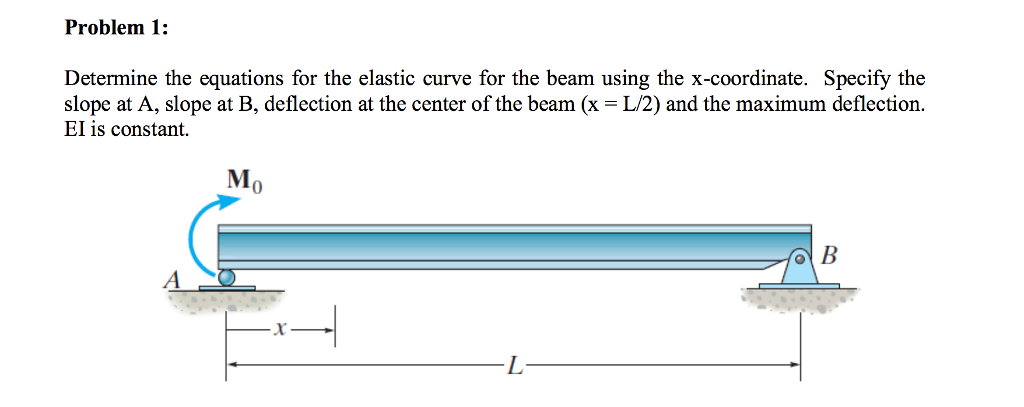# Problem 1:Determine the equations for the elastic curve for the beam using the x-coordinate. Specify theslope at A, slope at B, deflection at the center of the beam (x = L/2) and the maximum deflection.EI is constantMI0

Question
888 views
Determine the equations for the elastic curve for the beam using the x-coordinate. Specify the slope at A, slope at B, deflection at the center of the beam (x = L/2) and the maximum deflection. EI is constant.help_outlineImage TranscriptioncloseProblem 1: Determine the equations for the elastic curve for the beam using the x-coordinate. Specify the slope at A, slope at B, deflection at the center of the beam (x = L/2) and the maximum deflection. EI is constant MI 0 fullscreen
check_circle

Step 1

Determine the support reaction at A by taking moment about B.

Step 2

Determine the support reaction at B using the Equation of equilibrium.

Step 3

Determine the moment base...

### Want to see the full answer?

See Solution

#### Want to see this answer and more?

Solutions are written by subject experts who are available 24/7. Questions are typically answered within 1 hour.*

See Solution
*Response times may vary by subject and question.
Tagged in

### Structural Analysis